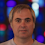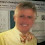## Monday, June 23, 2014

### How many ways can you make change of n cents?

(For a related post see  my post on Schur's theorem. The paper THIS post refers to is  here.)

(ADDED LATER: A commenter pointed to Graham-Knuth-Patashnik for a closed form for pennies, nickels, dimes, quarters, half-dollars. I have wriitten up their account and it is available here. I also apply their technique to just get 1,5,10,25.)

How many ways are there to make change of a dollar using pennies, nickels, dimes, and quarters? This is a well known question; however, the answers I found were disappointing. They were of two types
1. There are 242 ways to make change. The author then point to a program he wrote or to the actual list of ways to do it.
2. The number of ways to make n cents change is the coeff of x^n in the power series for 1/(1-x)(1-x^5)(1-x^{10})(1-x^{25}). This can be worked out.  I have never seen it worked out.
The first answer yields an actual number but is not interesting mathematically. The second answer is interesting mathematically but does not easily yield an actual number.

I looked for a closed form on the web but could not find one. So I did it myself. The paper  is pointed to above. I did not use generating functions, just recurrences. I do not know if it is new. Whether it is old or new I hope you enjoy it. The paper actually gives a closed form for the coin sets {1,x,kx} and {1,x,kx,rx}. Along the way we derive the answer for a dollar and the usual currency by hand.

This raises some questions:

1. Is my formula new? I'd be surprised either way. (Is it possible to be surprised at both statement A and statement NOT(A)?).  If  it's new I'll be surprised since the questions has been around for so long and surely someone would have done it. If it's known I'll be surprised since (a) I went to JSTOR and searched for all math journals they had for the words change'' and coin'', looked at the over 400 such articles (just the titles and abstracts) and didn't find it,  (b) this came up in math.stackexchange here and, true to form, every answer was either a program or a generating function and (c) other searches also did not turn up the result.(ADDED LATER- Since the 1,5,10,25,50 case was known, I guess I AM surprised.)
2. Even if its not new it is clearly not well known. Why is that?  Its a natural problem that people seemed to be interested in. One conjecture: The COMP SCI people interested in it were happy to write a program. The MATH people interested in it were happy to say `its merely the nth coeff of...' So a closed form solution seems to not be of interest to either camp
3. Is it interesting?  (a) Comp Sci: the closed form solution gives an answer in O(1) steps instead of the usual dynamic programming O(n) solution, and (b) Math: the closed form solution tells you the coefficients of the power series of 1/((1-x)(a-x^5)(1-x^{10})(1-x^{25}).

Side Bar: I asked the 10  students in my REU program to just GUESS how many ways there are to make change of a dollar with pennies, nickels, dimes, and quarters. Most made  guesses under 100. The lowest guess was 30. One person guessed 100! and one guessed 100!/2.  I then told them that it was between 30 and 100! and assigned them to derive it themselves by hand. Most were able to.

1.Regarding point 1. in the first list: the problem (with half-dollars) is worked out in Concrete Mathematics by Graham, Knuth, Pataschnik (page 330 in the 2nd ed.). The book also gives a closed form for this case later in that chapter.

1.Thanks! As soon as I got your comment I got a hold of GKP and looked at their proof and wrote it up and posted it as a link off of this blog.

2.Hmm. I’ll tell a little story on myself. The count-change program is given in the first edition of Abelson and Sussman, and a problem they give is to come up with a linear time algorithm for the problem. (Here, linear is in n, not log n.) It’s a stunning hard problem in context: early in a programming text — you can do it sort of like the iterative Fibonacci program, you just have to pass 50 or so variables.

About 20 years ago, it occurred to me that each of those 50 variable held a linear combination of the values they held at the previous iteration, so you could express evolution of the system as a matrix times a vector, and then use the fast-exponentiation algorithm on the matrix. This made the complexity logarithmic (in infinite precision arithmetic), and enabled me to evaluate the count-change function for huge numbers: 10^40th and thereabouts. And the results were interesting -- the numbers looked like they came out of context-free languages. Lots of regularities in the digits. So I showed this to Laci Babai, and a couple hours later, he came back with the answer via generating functions that consisted of 50 polynomials, one for each congruence class mod 50. [We used the version of the count change problem that included half-dollars.]

So the answer was "constant time," again, modulo infinite precision arithmetic.

3.Perhaps a Computational Complexity repost?

4.Do you know that coin change can be used to discover the anagrams, https://class.coursera.org/progfun-004/forum/thread?thread_id=1440?

5.You seem to have made an error under Def. 1.1 (Case 2) you stated a condition x > 2, but you hadn't previously defined x. Perhaps you meant s?

1.Also under Def. 2.2, number 4 ought to be d_n = c_n + d_n-u
(not d_n = b_n + cn_u)

2.THANKS! I fixed both in my copy--- I have much more to change as well, so the next version will have your corrections and others.

6.This file has appropriate code. I wrote it in grad school, longer ago than I care to think.

https://github.com/barak/oaklisp/blob/master/doc/examples/change.oak